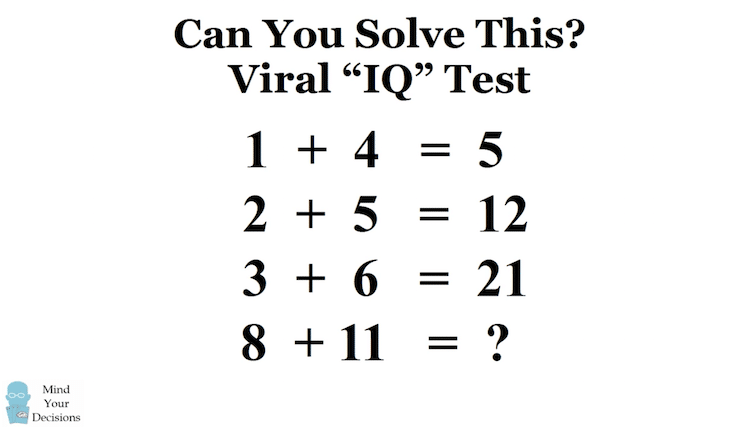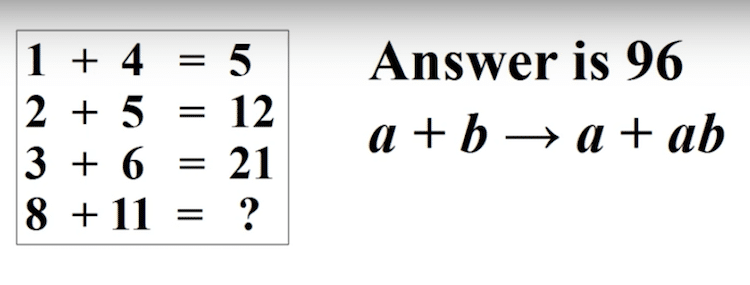# The Internet Can’t Seem to Agree on the Answer to This Math PuzzleDo you consider yourself a “puzzle person”? If so, take a shot at this math puzzle and see what you come up with. This deceptively simple puzzle went viral, with over 3 million people commenting on the IQ stumper. In fact, the original post declares that only one in a thousand people will actually figure it out.

Listen beautiful relax classics on our Youtube channel.

At this point, you may be thinking, “This puzzle doesn’t seem very difficult,” but you’d be wrong. Still don’t get it? Most people will default to using a running tally of numbers, where a + b = 5 and 5 + c +d = 12. This brings you to a final total of 40 when in reality, there’s another way to answer this brainbuster.Confused? It may be the first line that is throwing you off, as if we use the a + ab equation, 1 x 4 equals 4. However, in the following lines the equation becomes clearer. For instance, 2 + 2(5) equals 12. So when used in the last line, 8 + 8(11), the final answer is 96.

## If this math puzzle still isn’t clear, watch the full video explanation of this tricky IQ test.

And if that weren’t complicated enough, Presh Talwakar of MindYourDecisions also points out another pattern that some people see in the puzzle. Though rare, he states that “about 10 people saw this pattern out of 5+ million views this video has gotten,” it’s certainly another, complex way of solving things.

The idea is to evaluate the sums in base 10, and then convert the answer into descending number bases 6, 5, 4, etc. line by line. So the pattern is:

1 + 4 = 5 (base 10) = 5 (base 6)

2 + 5 = 7 (base 10) = 5*1 + 2 = 12 (base 5)

3 + 6 = 9 (base 10) = 4*2 + 1 = 21 (base 4)

So if we do the same for the last line, we would need to use base 3 to get:

8 + 11 = 19 (base 10) = 9*2 + 3*0 + 1 = 201 (base 3) So if you got 201, that’s another way people have seen the pattern.

h/t: [Shareably]